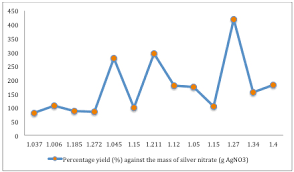## How to Calculate and Solve for Percentage Yield, Actual Yield and Theoretical Yield in Chemistry | The Calculator EncyclopediaThe image above represents percentage yield.

To compute the percentage yield, two essential parameters are needed and these parameters are actual yield and theoretical yield.

The formula for calculating percentage yield:

%Yield = (Actual YieldTheoretical Yield) x 100

Let’s solve an example;
Find the percentage yield when the actual yield is 12 with a theoretical yield of 26.

This implies that;
Actual yield = 12
Theoretical yield = 26

%Yield = (Actual YieldTheoretical Yield) x 100
%Yield = (1226) x 100
%Yield = 0.46 x 100
%Yield = 46.15

Therefore, the percentage yield is 46.15.

Calculating the Actual Yield when the Percentage Yield and the Theoretical Yield is Given.

Actual yield = %yield x theoretical yield / 100

Let’s solve an example;
Find the actual yield when the %yield is 52 with a theoretical yield of 10.

This implies that;
%yield = 52
Theoretical yield = 10

Actual yield = %yield x theoretical yield / 100
Actual yield = 52 x 10 / 100
Actual yield = 520 / 100
Actual yield = 5.2

Therefore, the actual yield is 5.2.